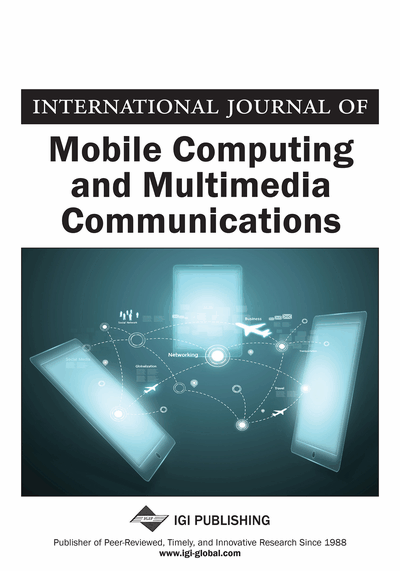# A Fast Image Encoding Algorithm Based on the Pyramid Structure of Codewords

Ahmed A. Radwan (Minia University, Egypt), Ahmed Swilem (Minia University, Egypt) and Mamdouh M. Gomaa (Minia University, Egypt)
DOI: 10.4018/jmcmc.2009072801

## Abstract

This article presents a very simple and efficient algorithm for codeword search in the vector quantization encoding. This algorithm uses 2-pixel merging norm pyramid structure to speed up the closest codeword search process. The authors first derive a condition to eliminate unnecessary matching operations from the search procedure. Then, based on this elimination condition, a fast search algorithm is suggested. Simulation results show that, the proposed search algorithm reduces the encoding complexity while maintaining the same encoding quality as that of the full search algorithm. It is also found that the proposed algorithm outperforms the existing search algorithms.
Article Preview
Top

## Introduction

A standard vector quantization (VQ) is an efficient data compression technique that has been widely applied to image and speech coding (Gray, 1984; Linde, Buzo, & Gray, 1980). A vector quantizer of rate r bits/sample and dimensionis a mapping from a-dimensional vector spaceinto some finite subset of it,where,. The subsetis called a codebook and its elements are called codewords or reproducing vectors. In the conventional full search method to encode an input vector, we have to find its distance from each ofcodewords, and then compare these distances to find the best-matched codeword.

A complete description of a vector quantization process includes three phases, namely training, encoding and decoding. The original signal is first segmented into individual vectors. The training phase is a codebook design procedure which tries to find the best set of representatives from a large set of input vectors using clustering algorithms like the generalized lioyd algorithm (GLA) (Gray, 1984; Linde, Buzo, & Gray, 1980). The GLA consists of alternatively applying the nearest neighbor condition and centroid condition to generate a sequence of codebooks with decreasing average distortion. The algorithm operates as follows: It begins with an initial codebook of size. It partitions the training set intosets of vectors using the nearest neighbor rule which associates each training vector with one of thecodeword’s in the codebook. This partitioning defines the vector encoder mapping. Then, a new codebook is created with the new codeword’s being the centroids of each of thepartition regions of input vectors. This new codebook defines the vector decoder mapping. The algorithm repeats these steps and calculates the average distortion for the new codebook over the entire training set at the end of each iteration. When the average distortion fails to decrease by a certain threshold amount, it is assumed that a local minimum in distortion has been reached and the algorithm terminates.

## Complete Article List

Search this Journal:
Reset
Open Access Articles: Forthcoming
Volume 11: 4 Issues (2020): Forthcoming, Available for Pre-Order
Volume 10: 4 Issues (2019)
Volume 9: 4 Issues (2018)
Volume 8: 4 Issues (2017)
Volume 7: 4 Issues (2016)
Volume 6: 4 Issues (2014)
Volume 5: 4 Issues (2013)
Volume 4: 4 Issues (2012)
Volume 3: 4 Issues (2011)
Volume 2: 4 Issues (2010)
Volume 1: 4 Issues (2009)
View Complete Journal Contents Listing